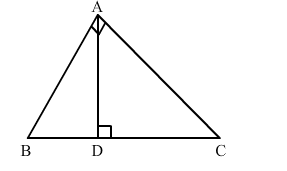# In the given figure ∠BAC = 90° and AD ⊥ BC. Then,Question:

In the given figure ∠BAC = 90° and AD ⊥ BC. Then,(a) BC ⋅ CD = BC2
(b) AB ⋅ AC = BC2
(c) BD ⋅ CD = AD2
(d) AB ⋅ AC = AD2

Solution:

(c) BD ⋅ CD = AD2

In $\triangle B D A$ and $\triangle A D C$, we have :

$\angle B D A=\angle A D C=90^{\circ}$

$\angle A B D=90^{\circ}-\angle D A B$

$=90^{\circ}-\left(90^{\circ}-\angle D A C\right)$

$=90^{\circ}-90^{\circ}+\angle D A C$

$=\angle D A C$

Applying $A A$ similarity theorem, we conclude that $\triangle B D A \sim \triangle A D C$.

$\Rightarrow \frac{B D}{A D}=\frac{A D}{C D}$

$\Rightarrow A D^{2}=B D \cdot C D$